# Standard Deviations & Capability Indices

Variation is present in everything we do. Indeed, no two things are exactly alike. One of the most widely accepted and quoted statistics for quantifying this variability is the standard deviation. However, there are many ways of calculating standard deviation and, therefore, different ways of applying process potential and performance indices. Estimates of standard deviation can be in the form of either short-term or long-term variation. The difference between the two is subtle, but important.

The short-term estimate of variability is often referred to as the process standard deviation or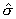(see Table1).

The long-term estimate of variation is referred to as the sample standard deviation or s (see Equation 1). Although these two standard deviation statistics each describe variation, their calculations, interpretation and use are vastly different.

### Short-Term Standard Deviation ()

If a control chart's range or s chart is in-control, it indicates that the variation from subgroup-to-subgroup is consistent and unchanging. The range and s statistics are both measures of within subgroup variation; a measure of short-term variability.

With variables data control charts, the standard deviation is estimated from different charts by using the formulas found in Table 1. Table 1's formulas are viable techniques for calculation of the short-term standard deviation () and for use with process capability indices Cp, Cpk, Cr, and Cpm (see Table 2).

ProFicient can calculateusing any of the formulas found in Table 1.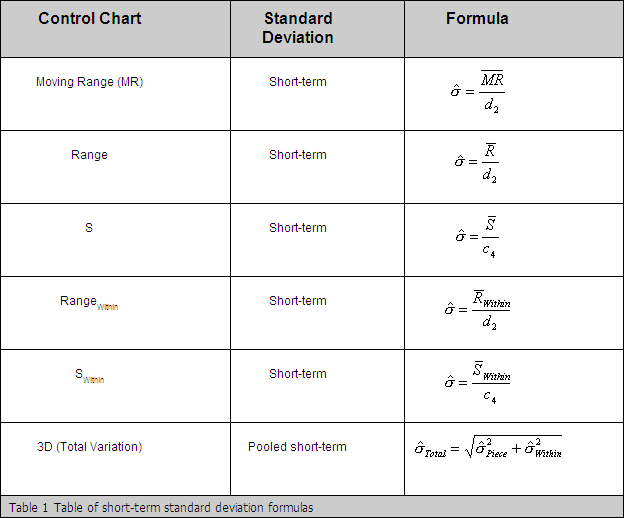### Long-Term Standard Deviation, s

The long-term standard deviation s, or sample standard deviation, is calculated in part by summing the differences between the individual data points and their data set's overall average (Equation 1). The inclusion of the overall average,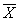, in the formula is what makes s considered to be a long-term estimate of variability, and it is the critical difference between short-term and long-term standard deviation.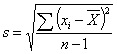Equation 1 Long-term estimate of variation, s

Long-term standard deviation, s, is used in calculating process performance indices like Pp, Ppk, Ppm, and Pr.

### What are the Differences Between s andTake a look at the control chart in Figure 1. The s chart is in-control, indicating that short-term variability is unchanging. However, thechart shows a distinct trend downward.  This downward trend is an example of the average changing over time, but any given snapshot in time (the range or s chart) is consistent over time.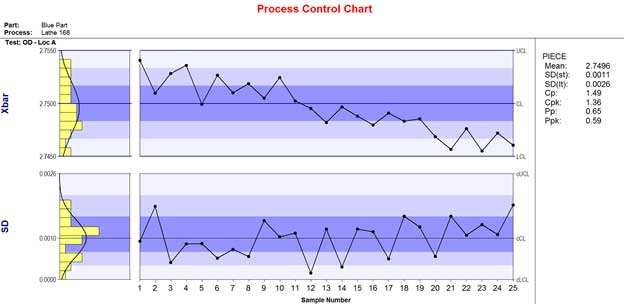Figure 1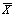-S chart with changes in long-term variability.

If bothand s charts remain consistent through time, the short-term and long-term estimates of variation will be approximately the same. If, however, the average changes significantly over time,will not include this variability sinceis calculated using only a short-term estimate of variability, (either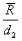or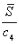). The process standard deviation,ignoresand any changes in its value. Therein lies the primary difference betweenand s.

### CP and CPK Explained

There also is an issue with the interpretation of the capability ratios. Sometimes Cp and Cpk values can be misleading. Note that the Cp and Cpk values on the right of the control chart are both larger than 1.33. This would typically indicate that although the trend exists, all products were produced within specification. However, this is not the case. The Pp ratio (calculated using the long-term estimate of variability, s) is equal to 0.65 while Ppk=0.59. Each of these indices is substantially less than the corresponding Cp and Cpk values, indicating the production of out-of-specification product. Since long-term variability is changing, the Pp and Ppk ratios more accurately represent the performance of the process.

If one is unaware of the underlying variability calculations in their capability ratios, erroneous data could be reported and costly decisions might be made.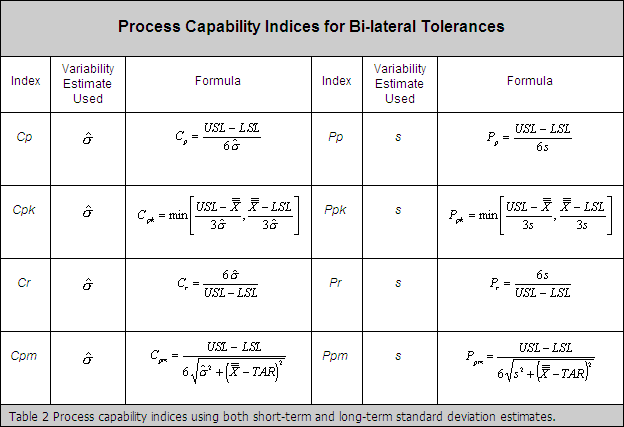Need help?
​For further information contact GetInTouch@infinityqs.com
Toll Free: 1.800.772.7978​

Take the first step from quality to excellence

### Take the first step from quality to excellence

Take the Next Steps